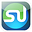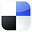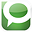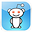# Not Normally Distributed

In the ideal world, all of your data samples are normally distributed. In this case you can usually apply the well-known parametric statistical tests such as ANOVA, the t Test, and regression to the sampled data.

What can you do if your data does not appear to be normally distributed?

You can either:

- Apply nonparametric tests to the data. Nonparametric tests do not rely on the underlying data to have any specific distribution

- Evaluate whether your “non-normal” data was really normally- distributed before it was affected by one of the seven correctable causes listed below:

The Biggest 7 Correctable Causes of Non-Normality in Data Samples

1) Outliers – Too many outliers can easily skew normally-distributed data. If you can identify and remove outliers that are caused by error in measurement or data entry, you might be able to obtain normally-distributed data from your skewed data set. Outliers should only be removed if a specific cause of their extreme value is identified. The nature of the normal distribution is that some outliers will occur. Outliers should be examined carefully if there are more than would be expected.

2) Data has been affected by more than one process – It is very important to understand all of the factors that can affect data sample measurement. Variations to process inputs might skew what would otherwise be normally-distributed output data. Input variation might be caused by factors such as shift changes, operator changes, or frequent changes in the underlying process. A common symptom that the output is being affected by more than one process is the occurrence of more than one mode (most commonly occurring value) in the output. In such a situation, you must isolate each input variation that is affecting the output. You must then isolate the overall effect which that variation had on the output. Finally, you must remove that input variation’s effect from output measurement. You may find that you now have normally-distributed data.

3) Not enough data – A normal process will not look normal at all until enough samples have been collected. It is often stated that 30 is the where a “large” sample starts. If you have collected 50 or fewer samples and do not have a normally-distributed sample, collect at least 100 samples before re-evaluating the normality of the population from which the samples are drawn.

4) Measuring devices that have poor resolution – Devices with poor resolution may round off incorrectly or make continuous data appear discrete. You can, of course, use a more accurate measuring device. A simpler solution is to use a much larger sample size to smooth out sharp edges.

5) A different distribution describes the data – Some forms of data inherently follow different distributions. For example, radioactive decay is described by the exponential distribution. The Poisson distribution describes events event that tend to occur at predictable intervals over time, such as calls over a switchboard, number of defects, or demand for services. The lengths of time between occurrences of Poisson-distributed processes are described by the exponential distribution. The uniform distribution describes events that have an equal probability of occurring. Application of the Gamma distribution often based on intervals between Poisson-distributed events, such as queuing models and the flow of items through a manufacturing process. The Beta distribution is often used for modeling planning and control systems such are PERT and CPM. The Weibull distribution is used extensively to model time between failure of manufactured items, finance, and climatology. It is important to become familiar with the applications of other distributions. If you know that the data is described by a different distribution than the normal distribution, you will have to apply the techniques of that distribution or use nonparametric analysis techniques.

6) Data approaching zero or a natural limit – If the data has a large number of value than are near zero or a natural limit, the data may appear to be skewed. In this case, you may have to adjust all data by adding a specific value to all data being analyzed. You need to make sure that all data being analyzed is “raised” to the same extent.

7) Only a subset of process’ output is being analyzed – If you are sampling only a specific subset of the total output of a process, you are likely not collecting a representative sample from the process and therefore will not have normally distributed samples. For example, if you are evaluating manufacturing samples that occur between 4 and 6AM and not an entire shift, you might not obtain the normally-distributed sample that a whole shift would provide. It is important to ensure that your sample is representative of an entire process.

If you are unable to obtain a normally-distributed data sample, you can usually apply non-parametric tests to the data.

If you would like to create a link to this blog article, here is the link to copy for your convenience:

Correctable Reasons Why Your Data Is Not Normally DistributedIf You Like This, Then Share It...Excel Master Series Blog Directory

Statistical Topics and Articles In Each Topic

• Histograms in Excel
• Bar Chart in Excel
• Combinations & Permutations in Excel
• Normal Distribution in Excel
• t-Distribution in Excel
• Binomial Distribution in Excel
• z-Tests in Excel
• t-Tests in Excel
• Hypothesis Tests of Proportion in Excel
• Chi-Square Independence Tests in Excel
• Chi-Square Goodness-Of-Fit Tests in Excel
• F Tests in Excel
• Correlation in Excel
• Pearson Correlation in Excel
• Spearman Correlation in Excel
• Confidence Intervals in Excel
• Simple Linear Regression in Excel
• Multiple Linear Regression in Excel
• Logistic Regression in Excel
• Single-Factor ANOVA in Excel
• Two-Factor ANOVA With Replication in Excel
• Two-Factor ANOVA Without Replication in Excel
• Creating Interactive Graphs of Statistical Distributions in Excel
• Solving Problems With Other Distributions in Excel
• Optimization With Excel Solver
• Chi-Square Population Variance Test in Excel
• Analyzing Data With Pivot Tables
• SEO Functions in Excel
• Time Series Analysis in Excel

1.2.